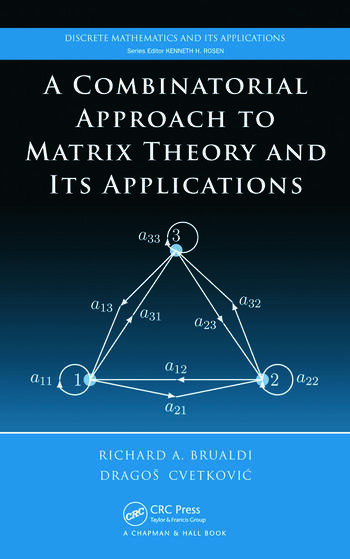# A Combinatorial Approach to Matrix Theory and Its Applications

## 1st Edition

Richard A. Brualdi, Dragos Cvetkovic

Chapman and Hall/CRC
Published August 6, 2008
Reference - 288 Pages - 44 B/W Illustrations
ISBN 9781420082234 - CAT# C8223
Series: Discrete Mathematics and Its Applications

For Instructors Request Inspection Copy

USD\$135.00

FREE Standard Shipping!

## Preview

### Summary

Unlike most elementary books on matrices, A Combinatorial Approach to Matrix Theory and Its Applications employs combinatorial and graph-theoretical tools to develop basic theorems of matrix theory, shedding new light on the subject by exploring the connections of these tools to matrices.

After reviewing the basics of graph theory, elementary counting formulas, fields, and vector spaces, the book explains the algebra of matrices and uses the König digraph to carry out simple matrix operations. It then discusses matrix powers, provides a graph-theoretical definition of the determinant using the Coates digraph of a matrix, and presents a graph-theoretical interpretation of matrix inverses. The authors develop the elementary theory of solutions of systems of linear equations and show how to use the Coates digraph to solve a linear system. They also explore the eigenvalues, eigenvectors, and characteristic polynomial of a matrix; examine the important properties of nonnegative matrices that are part of the Perron–Frobenius theory; and study eigenvalue inclusion regions and sign-nonsingular matrices. The final chapter presents applications to electrical engineering, physics, and chemistry.

Using combinatorial and graph-theoretical tools, this book enables a solid understanding of the fundamentals of matrix theory and its application to scientific areas.

#### Instructors

We provide complimentary e-inspection copies of primary textbooks to instructors considering our books for course adoption.